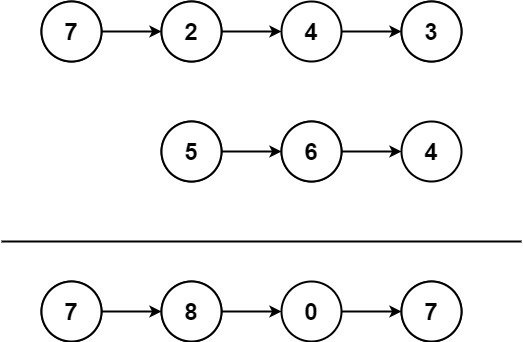### LeetCode• ㊗️
• 大家
• offer
• 多多！

## Problem

You are given two non-empty linked lists representing two non-negative integers. The most significant digit comes first and each of their nodes contains a single digit. Add the two numbers and return the sum as a linked list.

You may assume the two numbers do not contain any leading zero, except the number 0 itself.

Example 1:Input: l1 = [7,2,4,3], l2 = [5,6,4]
Output: [7,8,0,7]


Example 2:

Input: l1 = [2,4,3], l2 = [5,6,4]
Output: [8,0,7]


Example 3:

Input: l1 = , l2 = 
Output: 


## Code

/**
* public class ListNode {
*     int val;
*     ListNode next;
*     ListNode() {}
*     ListNode(int val) { this.val = val; }
*     ListNode(int val, ListNode next) { this.val = val; this.next = next; }
* }
*/
class Solution {
public ListNode addTwoNumbers(ListNode l1, ListNode l2) {
int len1 = 0;
int len2 = 0;
ListNode curr1 = l1;
ListNode curr2 = l2;

while (curr1 != null) {
curr1 = curr1.next;
len1++;
}

while (curr2 != null) {
curr2 = curr2.next;
len2++;
}

// 3->3->3 + 7->7 => 10->10->3
curr1 = l1;
curr2 = l2;

while (len1 > 0 && len2 > 0) {
int sum = 0;

if (len1 >= len2) {
sum += curr1.val;
curr1 = curr1.next;
len1--;
}

if (len1 < len2) {
sum += curr2.val;
curr2 = curr2.next;
len2--;
}

ListNode curr = new ListNode(sum);
}

// 10->10->3 --> 0->1->4 --> 4->1->0
int carry = 0;

while (curr != null) {
int sum = curr.val + carry;

int val = sum % 10;
carry = sum / 10;

ListNode temp = new ListNode(val);
curr = curr.next;
}

if (carry != 0) {
ListNode temp = new ListNode(carry);
}

}
}

/**
* public class ListNode {
*     int val;
*     ListNode next;
*     ListNode() {}
*     ListNode(int val) { this.val = val; }
*     ListNode(int val, ListNode next) { this.val = val; this.next = next; }
* }
*/
class Solution {
public ListNode addTwoNumbers(ListNode l1, ListNode l2) {
ListNode l1Rev = reverse(l1);
ListNode l2Rev = reverse(l2);

ListNode dummy = new ListNode();
ListNode curr = dummy;

int carry = 0;
while(l1Rev != null || l2Rev != null) {
int num1 = l1Rev == null ? 0 : l1Rev.val;
int num2 = l2Rev == null ? 0 : l2Rev.val;

int sum = num1 + num2 + carry;
carry = sum / 10;
int remain = sum % 10;
curr.next = new ListNode(remain);
curr = curr.next;

if(l1Rev != null) l1Rev = l1Rev.next;
if(l2Rev != null) l2Rev = l2Rev.next;
}

if(carry != 0) {
curr.next = new ListNode(carry);
}

return reverse(dummy.next);
}